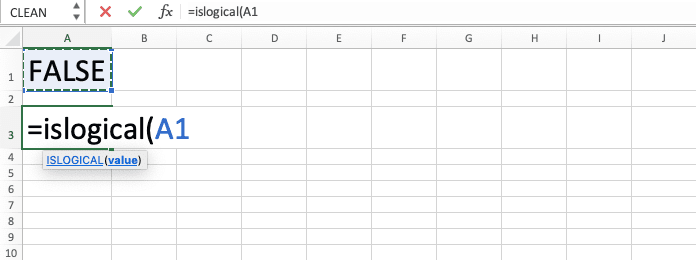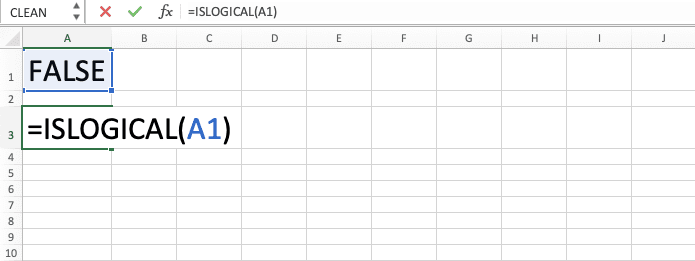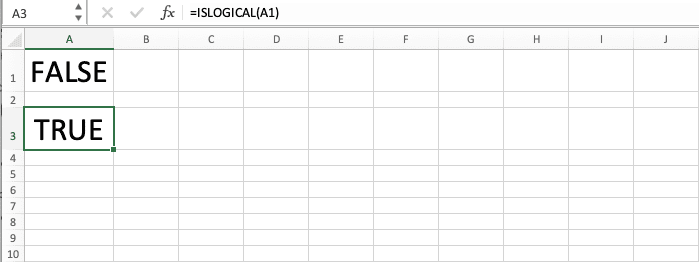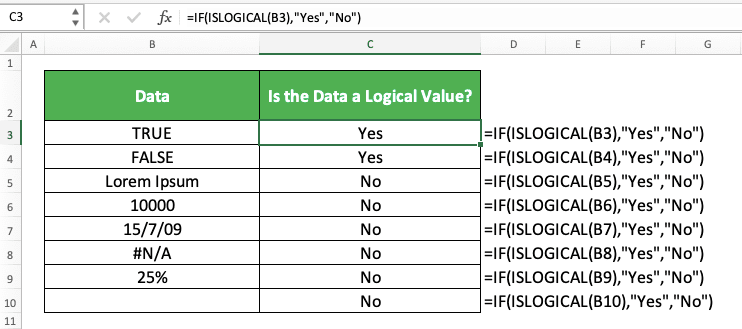How to Use the ISLOGICAL Function in Excel: Usabilities, Examples, and Writing Steps - Compute Expert

# How to Use the ISLOGICAL Function in Excel: Usabilities, Examples, and Writing Steps

Home >> Excel Tutorials from Compute Expert >> Excel Formulas List >> How to Use the ISLOGICAL Function in Excel: Usabilities, Examples, and Writing Steps

In this tutorial, you will learn how to use the ISLOGICAL function in excel completely.

When working in excel, we may want to check whether our data is a logical value (TRUE or FALSE) or not. If we use ISLOGICAL, then we can do this checking process easily and fast.

Want to learn more about ISLOGICAL and master the way to use it in excel? Read this Compute Expert tutorial until its last part!

Disclaimer: This post may contain affiliate links from which we earn commission from qualifying purchases/actions at no additional cost for you. Learn more

## What is the ISLOGICAL Function in Excel?

ISLOGICAL is an excel function that helps you to identify whether your data is a logical value or not.

## ISLOGICAL Usability

You can use ISLOGICAL to check whether your data is a logical value or not. You will get a TRUE or FALSE depending on the checking result.

## ISLOGICAL Result

The ISLOGICAL result is a TRUE or FALSE logical value. The one you get depends on whether your data is a logical value (TRUE) or not (FALSE).

## Excel Version from Which We Can Start Using ISLOGICAL

We can start using ISLOGICAL in excel since excel 2003.

## The Way to Write It and Its Input

Here is the general writing form of ISLOGICAL in excel.

= ISLOGICAL ( data )

When writing ISLOGICAL, you just need to input the data you want to check with the formula. Besides data, you can also input a formula to check whether the formula result is a logical value or not.

## Example of Its Usage and Input

Here is an implementation example of ISLOGICAL in excel.As you can see in the example, it is quite easy to implement ISLOGICAL in excel. Just input the data/formula you want to check with ISLOGICAL and press enter. You will immediately get your result!

You can also see in the example that ISLOGICAL only gives TRUE if its input value is TRUE or FALSE. It gives FALSE for other things like text, number, date, empty cell, or even an error.

## Writing Steps

Need guidance when you write your ISLOGICAL formula in excel? See these ISLOGICAL writing steps below!

1. Type an equal sign ( = ) in the cell where you want to put your ISLOGICAL result2. Type ISLOGICAL (can be with large and small letters) and an open bracket sign after =3. Input the data/formula you want to check with ISLOGICAL. ISLOGICAL will only produce TRUE if your data/formula has a logical value (TRUE or FALSE)4. Type a close bracket sign5. Press Enter
6. Done!## Check if Our Data isn’t a Logical Value: NOT ISLOGICAL

Need to get a TRUE if your data isn’t a logical value instead? You can combine ISLOGICAL with a NOT function for that purpose!

NOT is an excel function you can use to overturn TRUE to FALSE and FALSE to TRUE. By combining it with ISLOGICAL, you can get TRUE if the data you input into ISLOGICAL isn’t a logical value!

Here is the general writing form of the NOT and ISLOGICAL combination in excel.

= NOT ( ISLOGICAL ( data ) )

Just input your ISLOGICAL writing in the NOT formula you write. Don’t forget to input the data you want to check inside the ISLOGICAL.

Here is the two formulas combination implementation example in excel for a better understanding of its concept.As you can see there, we can get a TRUE for a non-logical value and FALSE for a logical value. That is because we combine NOT and ISLOGICAL in the way we have discussed!

## Get a Particular Result if Our Data is a Logical Value: IF ISLOGICAL

Need to get a result other than TRUE or FALSE from your data checking process with ISLOGICAL? To get that kind of result, you can combine your ISLOGICAL writing with an IF.

Here is the general writing form of the IF and ISLOGICAL combination in excel for this purpose.

= IF ( ISLOGICAL ( data ) , result_if_logical_value , result_if_not_logical_value )

We write our ISLOGICAL in the logic condition input part of the IF. After that, we input the result we want from the IF if the ISLOGICAL produces TRUE or FALSE.

By doing that, we can get the result we want if the data we check is a logical value or not!

Here is the implementation example of our IF and ISLOGICAL combination in excel.In this example, we can get a “Yes” and “No” result from our data identification process with ISLOGICAL. That is because we combine our ISLOGICAL writing with an IF.

Whenever you need a result other than TRUE or FALSE from ISLOGICAL, combine it with an IF!

## Exercise

After you have learned how to use ISLOGICAL in excel completely, now let’s do an exercise to deepen your understanding.

### Instructions

Answer each question in the gray-colored cell on the appropriate row. Use ISLOGICAL (with an IF if appropriate) to get your answer for each question!
1. Is the left cell contains a logical value? Use TRUE or FALSE to answer!
2. Is the left cell contains a logical value? Answer with “V” if yes or “X” if not!
3. Is the left cell contains a logical value? Assume that 1 is TRUE and 0 is FALSE and answer with “Yes” if yes or “No” if not! See the additional note section to get a clue on how to answer this question!

Even though TRUE is equal to 1 and FALSE is equal to 0 in excel, ISLOGICAL doesn’t treat them that way. It will produce FALSE if you try to input 1 or 0 to it.If you need to treat 1 as TRUE or 0 as FALSE in ISLOGICAL, you can apply AND or OR beforehand. That way, the 1 or 0 will turn into TRUE or FALSE and ISLOGICAL will give you a TRUE!Related tutorials you should learn too:

Get updated excel info from Compute Expert by registering your email. It's free!